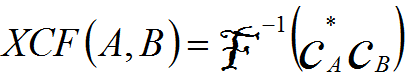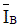Cross Correlation Function (XCF)/Coefficient between Two Images
- Practical Electron Microscopy and Database -
- An Online Book -

https://www.globalsino.com/EM/

 ================================================================================= The cross correlation between two images can be evaluated by cross-correlation function (XCF) or cross-correlation coefficient (XCC). The Cross Correlation Function (XCF) between any two images Cx(r) and Cy(r)  can be defined in terms of their Fourier transforms, given by,--------------------- [3717a] The Cross Correlation Coefficient between the two images can be defined as,--------------------- [3717b] where,and-- The mean values of the image IA and IB, respectively.           x and y -- The coordinates in the two images (e.g. the coordinates of a pixel if the images are in digital format). From Equation 3717b, we can know the coefficient XCC(A,B) is equal to 1 if the two images are absolutely identical. In the case of which Gatan DM is used, XCC(A,B) based on Equation 3717b can be computed by scripts.
=================================================================================